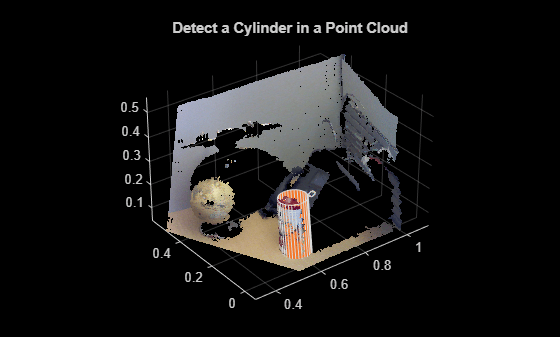# cylinderModel

Parametric cylinder model

## Description

The `cylinderModel` object stores a parametric cylinder model.

## Creation

### Syntax

``model = cylinderModel(params)``

### Description

example

````model = cylinderModel(params)` constructs a parametric cylinder model from the specified `params`. The `params` argument is a 1-by-7 numeric vector that determines the value of the `Parameters` property.```

## Properties

expand all

Cylinder model parameters, stored as a 1-by-7 numeric vector. The vector is of the form [x1 y1 z1 x2 y2 z2 r].

• [x1 y1 z1] and [x2 y2 z2] are the 3-D centers of each end-cap surface of the cylinder.

• r is the radius of the cylinder.

Center of the cylinder, stored as a 1-by-3 numeric vector representing the x-, y-, and z-coordinates.

Height of the cylinder, stored as a scalar.

Radius of the cylinder, stored as a scalar.

## Object Functions

 `plot` Plot parametric model

## Examples

collapse all

Load a MAT file containing a point cloud into the workspace.

`load("object3d.mat");`

Display the point cloud.

```figure pcshow(ptCloud) xlabel("X(m)") ylabel("Y(m)") zlabel("Z(m)") title("Detect a Cylinder in a Point Cloud")```

Set the maximum point-to-cylinder distance for cylinder fitting to 5mm.

`maxDistance = 0.005;`

Specify a region of interest (ROI) to constrain the fitting function.

```roi = [0.4 0.6; -inf 0.2; 0.1 inf]; sampleIndices = findPointsInROI(ptCloud,roi);```

Set the orientation constraint for the fitting function

`referenceVector = [0 0 1];`

Detect the cylinder in the specified ROI of the point cloud and extract it.

```model = pcfitcylinder(ptCloud,maxDistance,referenceVector, ... SampleIndices=sampleIndices);```

Plot the model of the detected cylinder.

```hold on plot(model)```## Version History

Introduced in R2015b